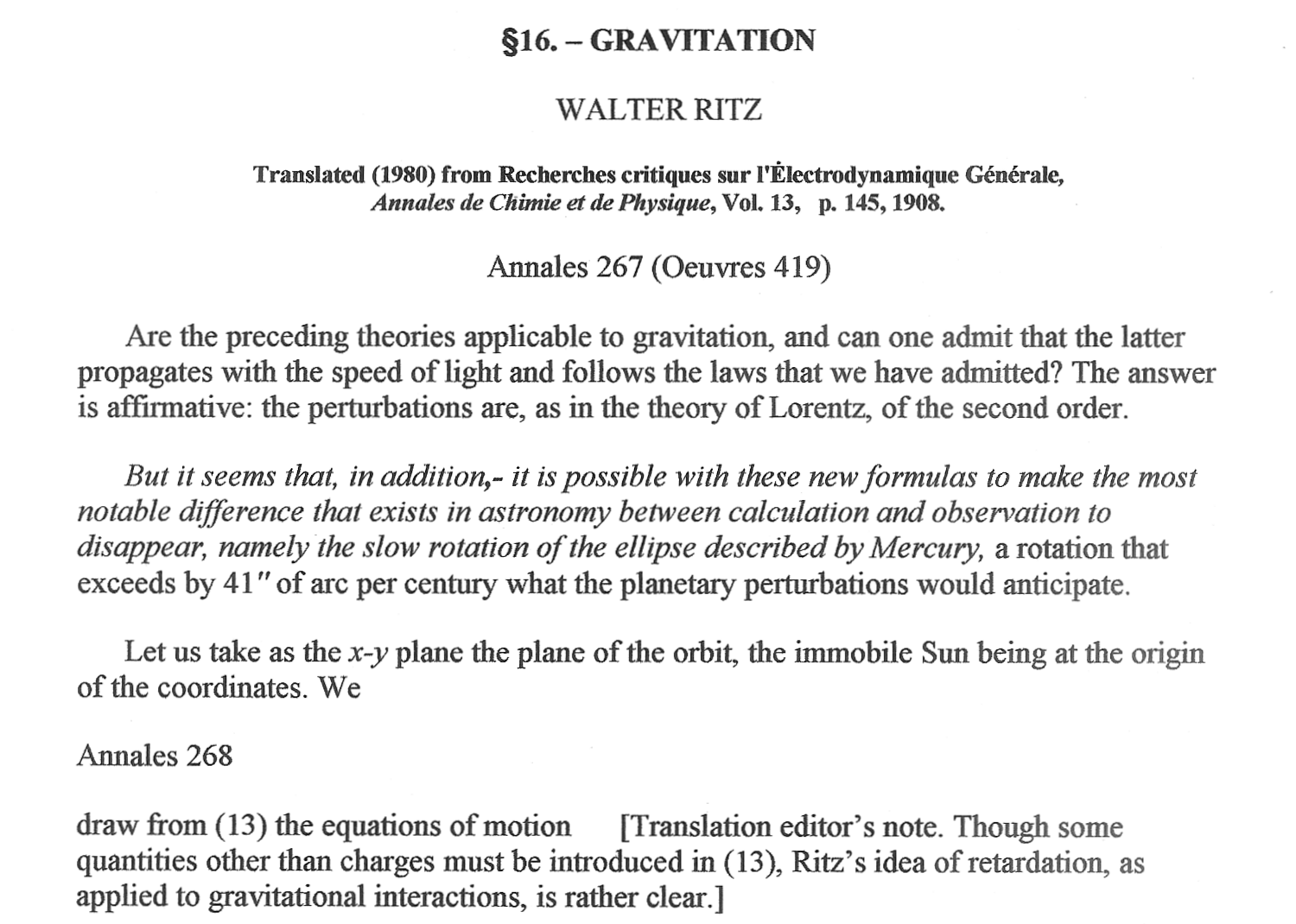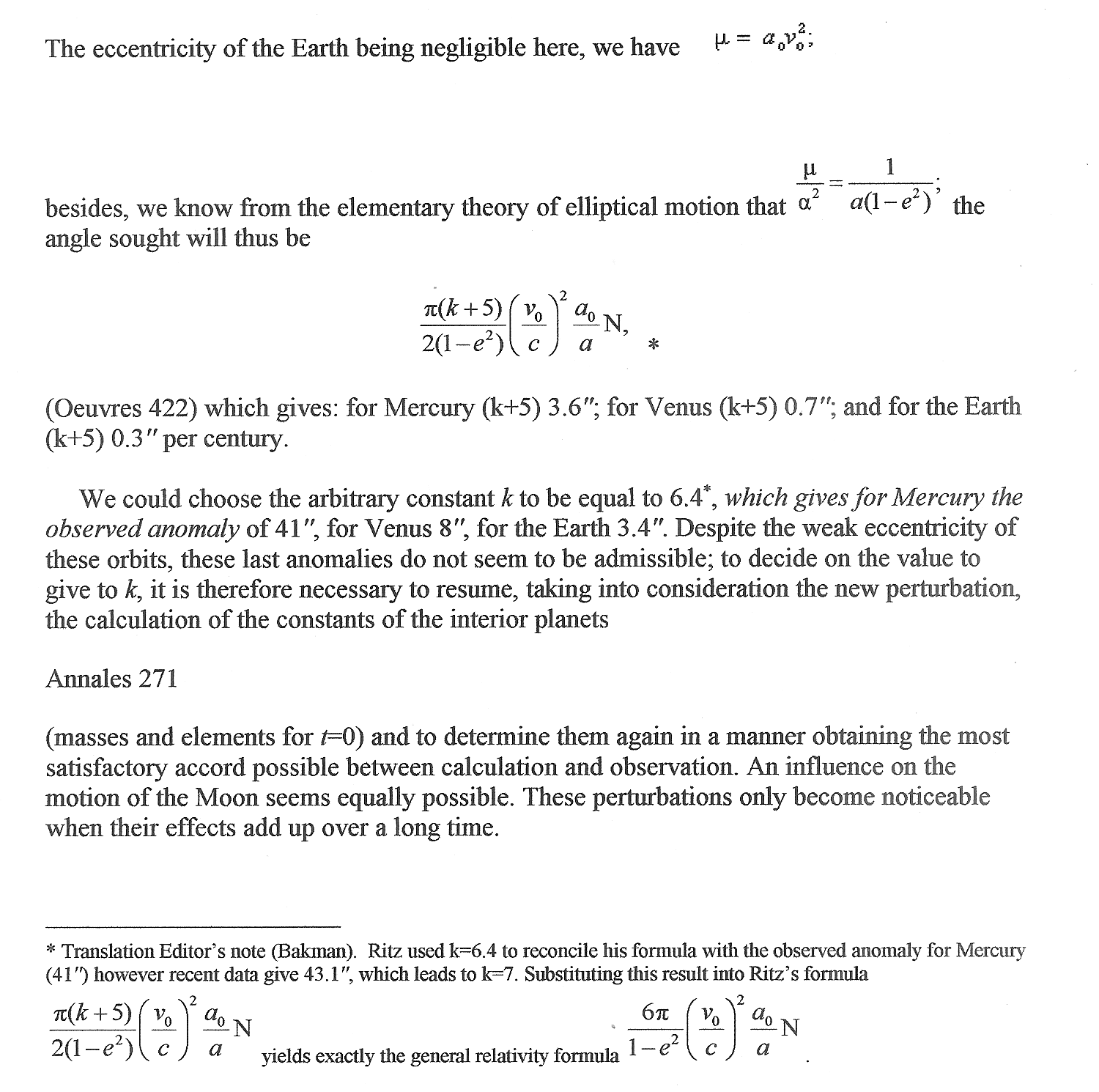﻿ GRAVITATION
Home | Previous | Up one level | Next[Additional translation editor's note (Bakman): I think that (13), above, is equation (13) from §3. Ritz's assumption was that the gravitational equations are similar to those of electrodynamics. The difference is that (52) was written for acceleration instead of force in (13), also μ in (52) is equal to G*Msun and cos(r,x)=x/r. 08 Jan 2017.]Table of Contents | Previous Section | Next Section Home | Previous | Up one level | Next